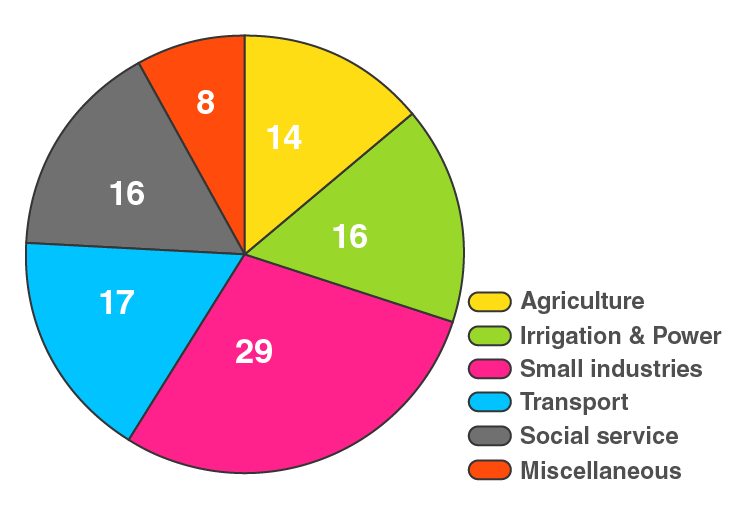# RD Sharma Solutions for Class 8 Maths Chapter 25 Data Handling - III (Pictorial Representation of Data as Pie Charts or Circle Graphs) Exercise 25.1

In Exercise 25.1 of Chapter 25, we shall discuss concepts related to pie-diagrams or pie-charts and construction of pie-diagrams. Students having any difficulties in solving the problems can access RD Sharma Class 8 Solutions. Our experts have solved the problems in a simple and easily understandable manner, which any student can understand and thereby, score well in their exams. To access the solutions of RD Sharma Class 8 Maths Chapter 25, students can download the pdf from the links given below.

## Download the Pdf of RD Sharma Solutions for Class 8 Maths Exercise 25.1 Chapter 25 Data Handling – III (Pictorial Representation of Data as Pie Charts or Circle Graphs)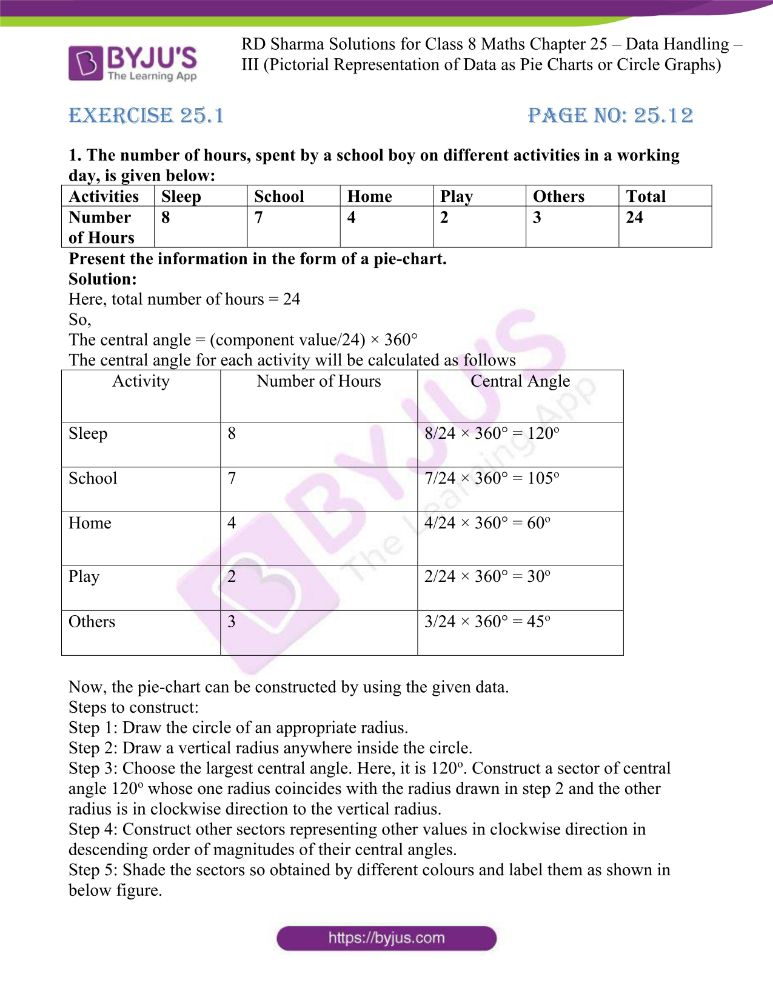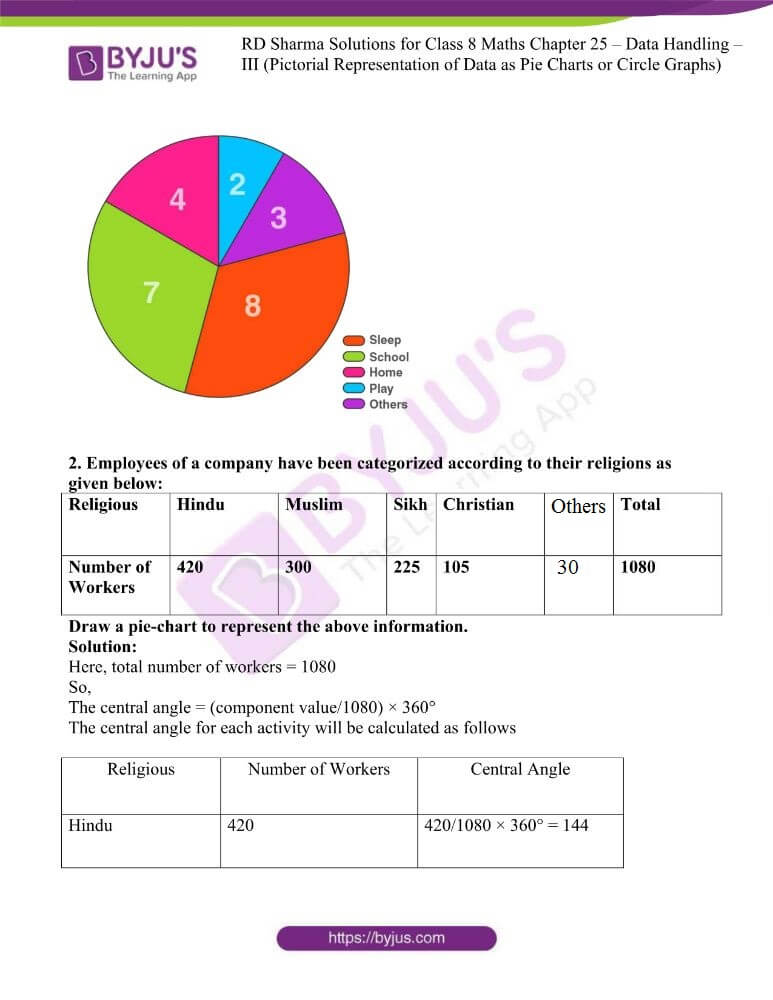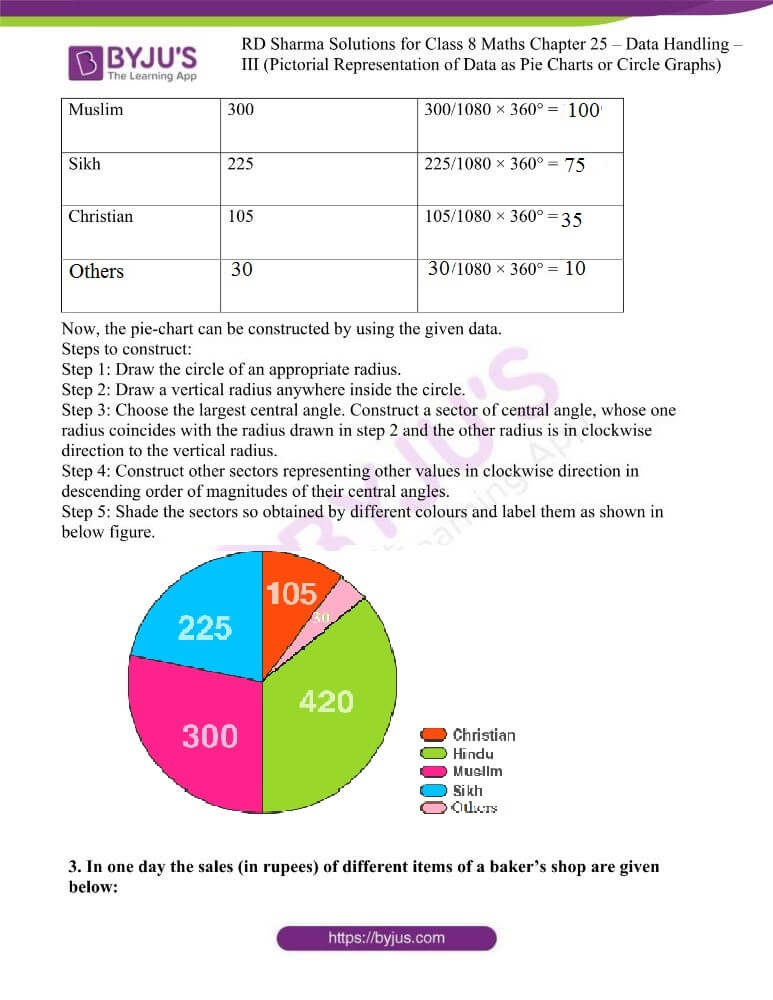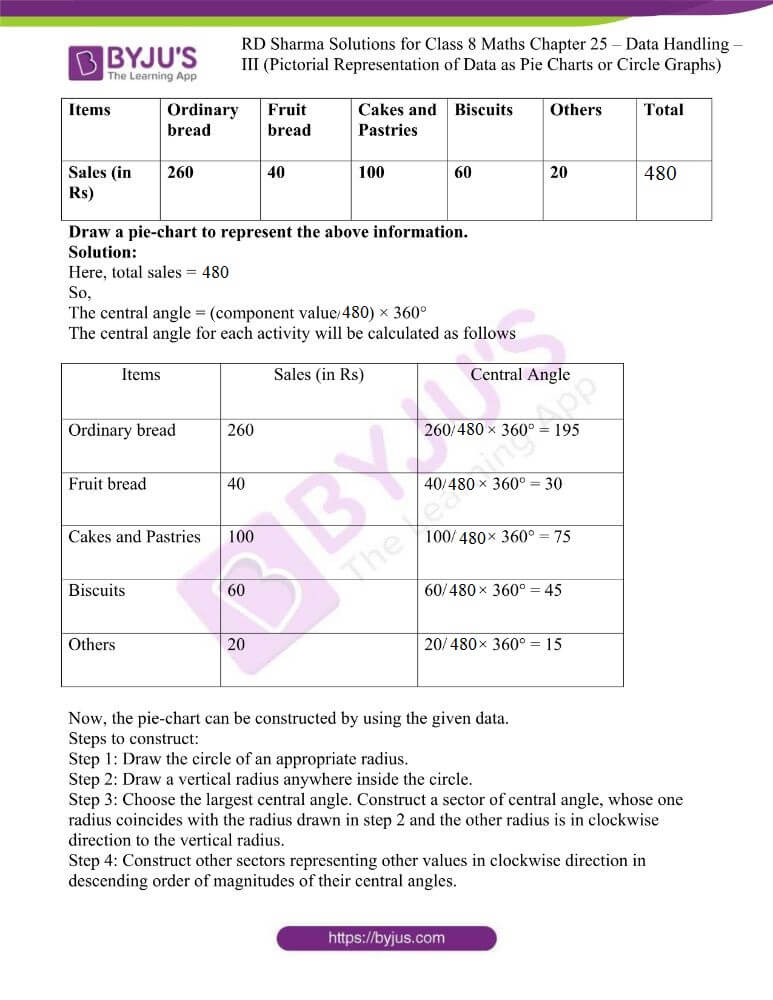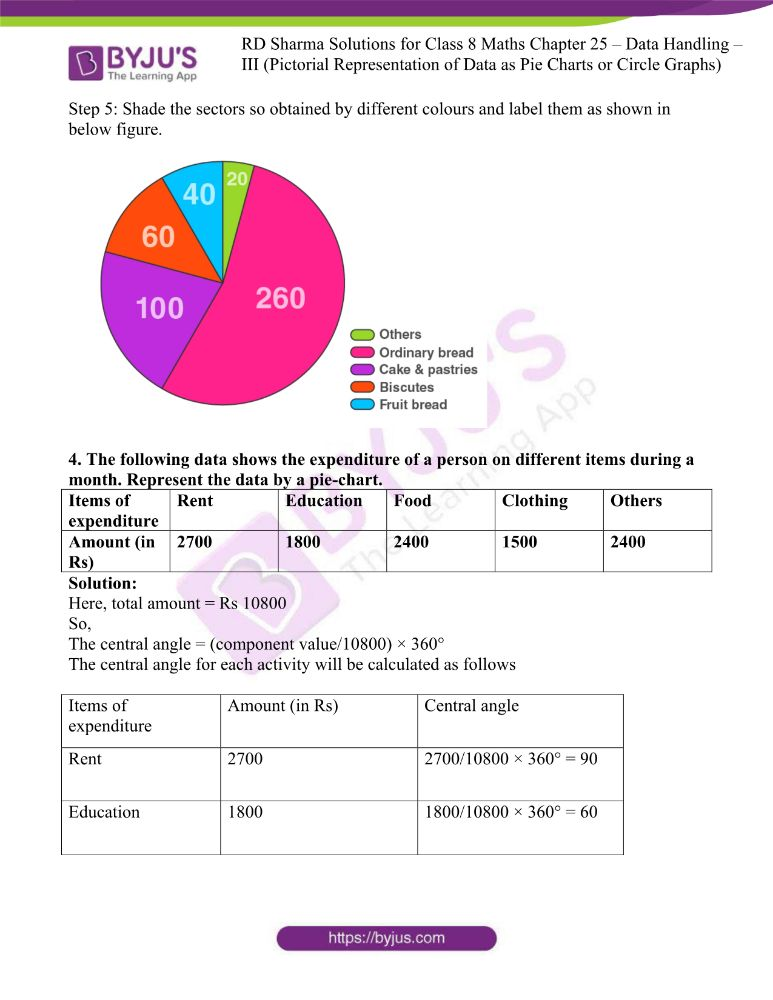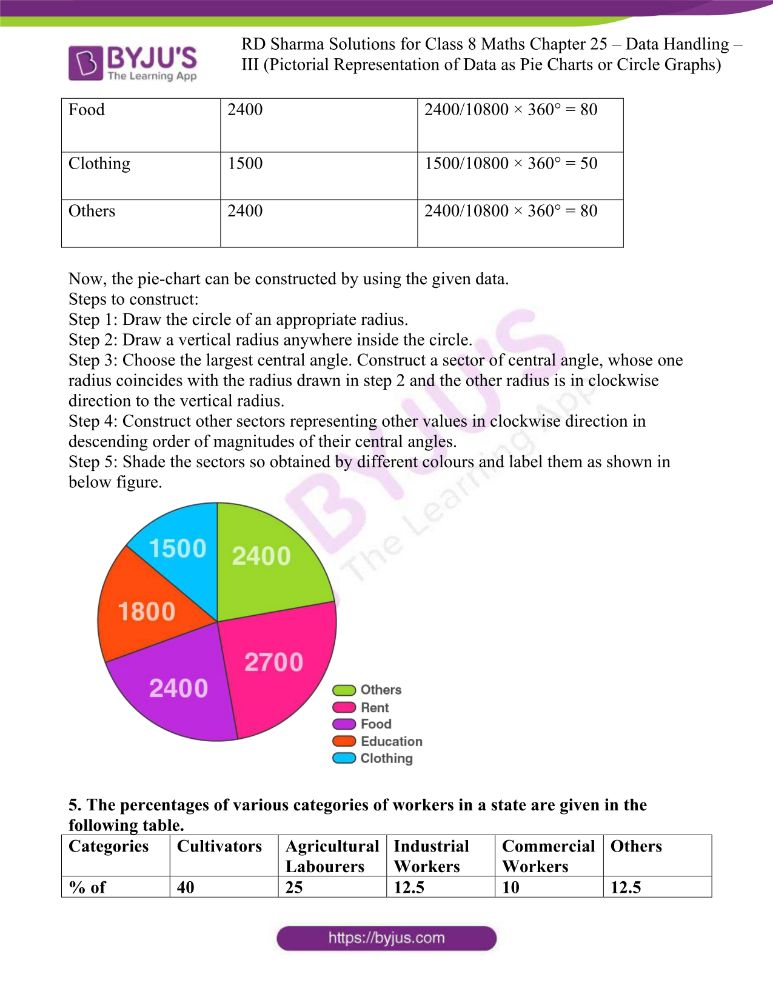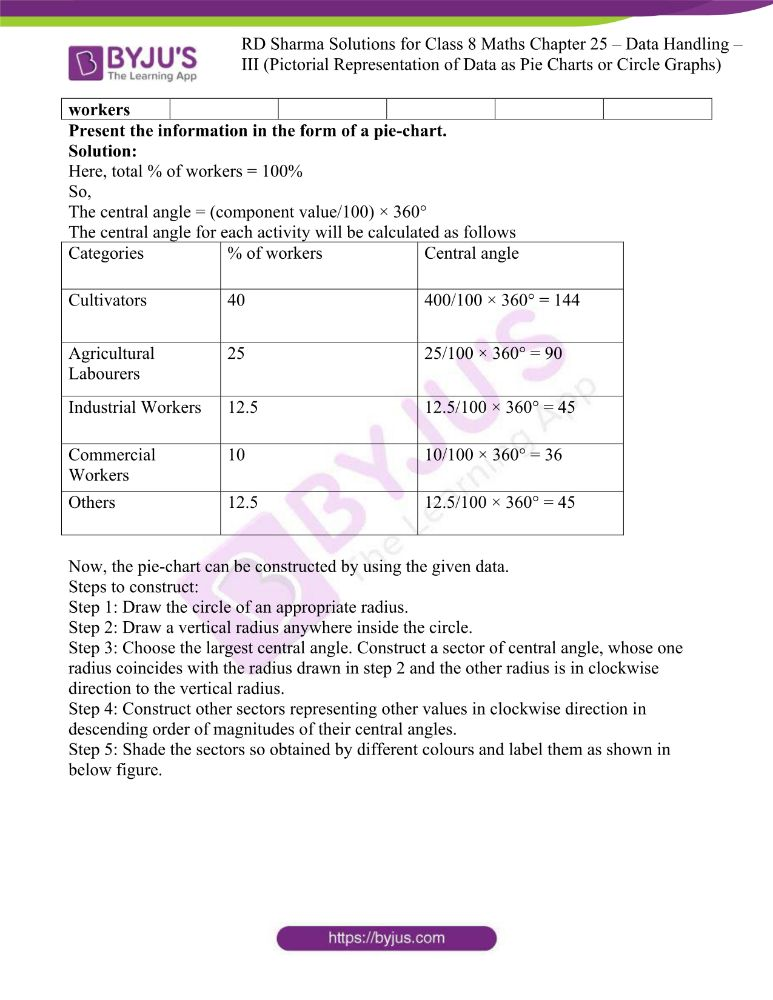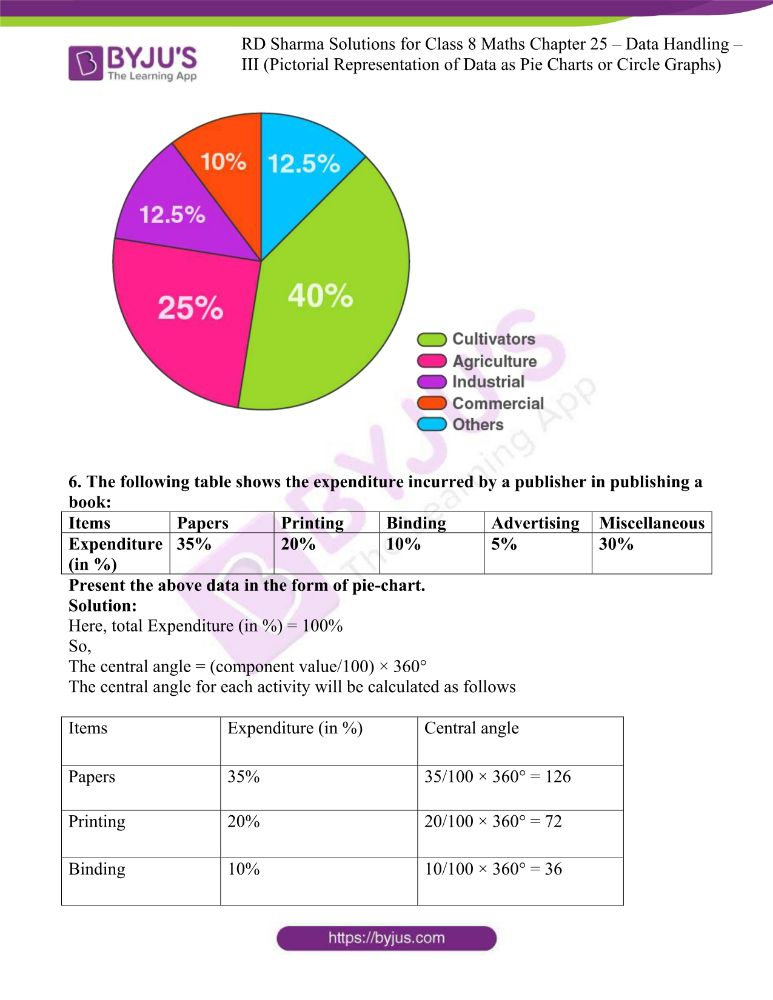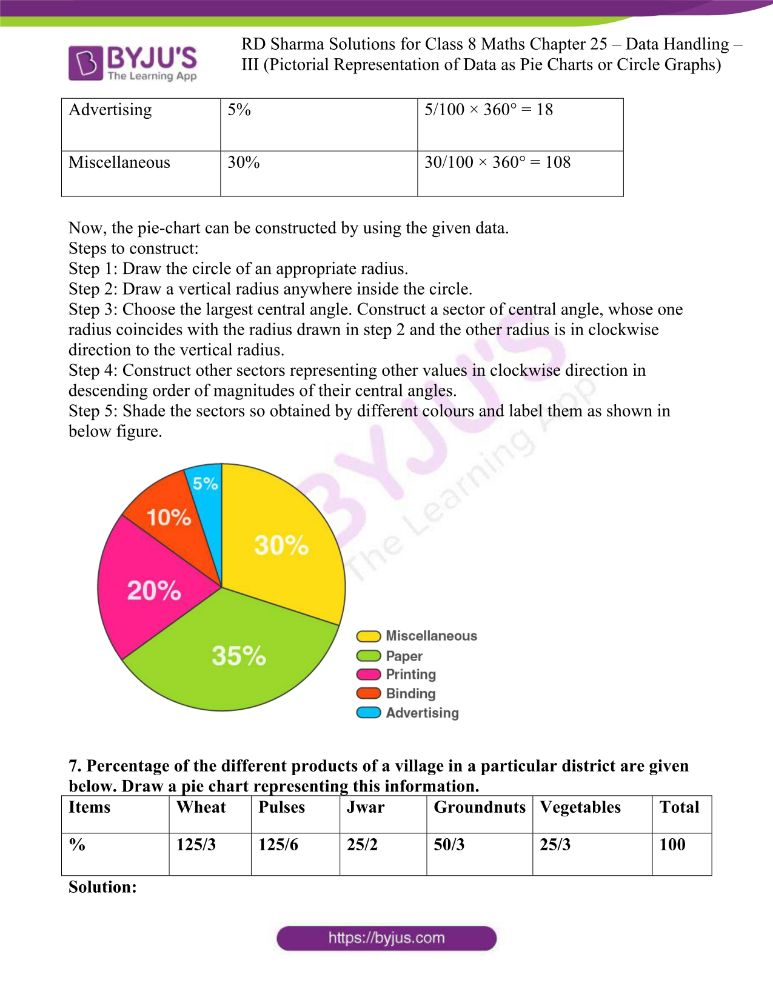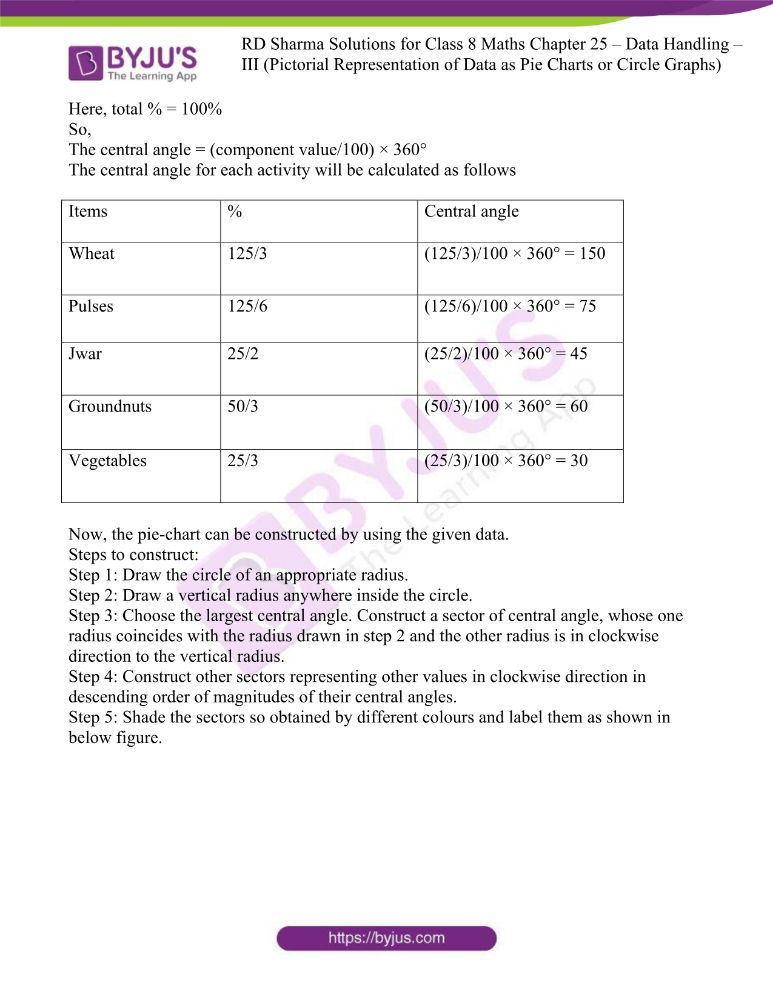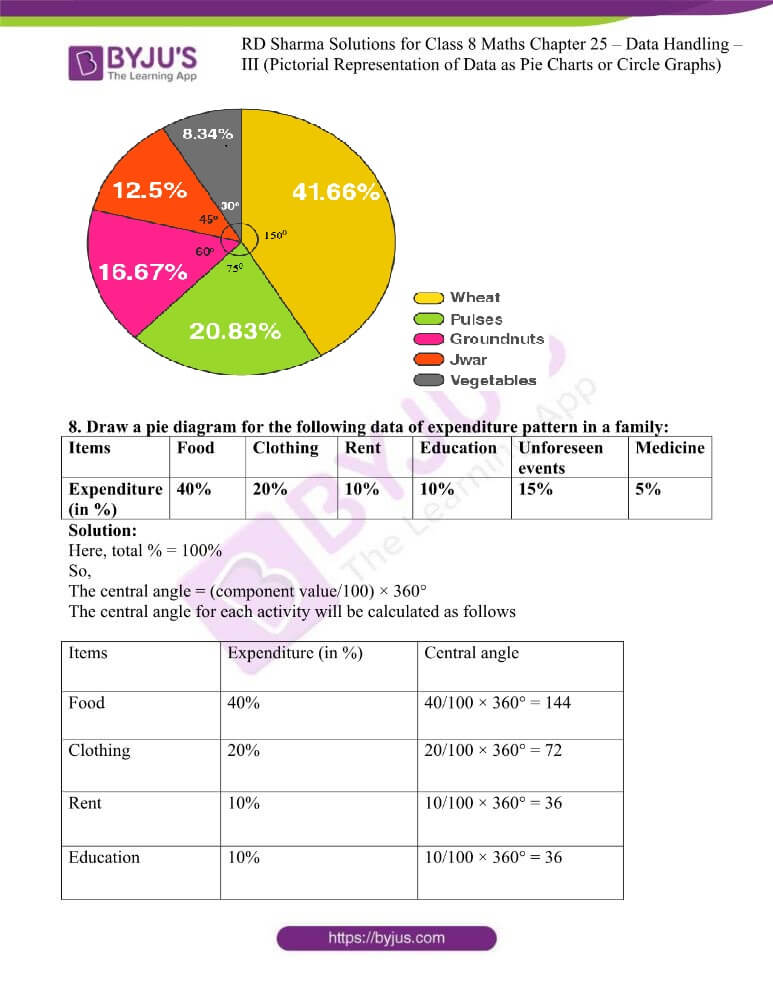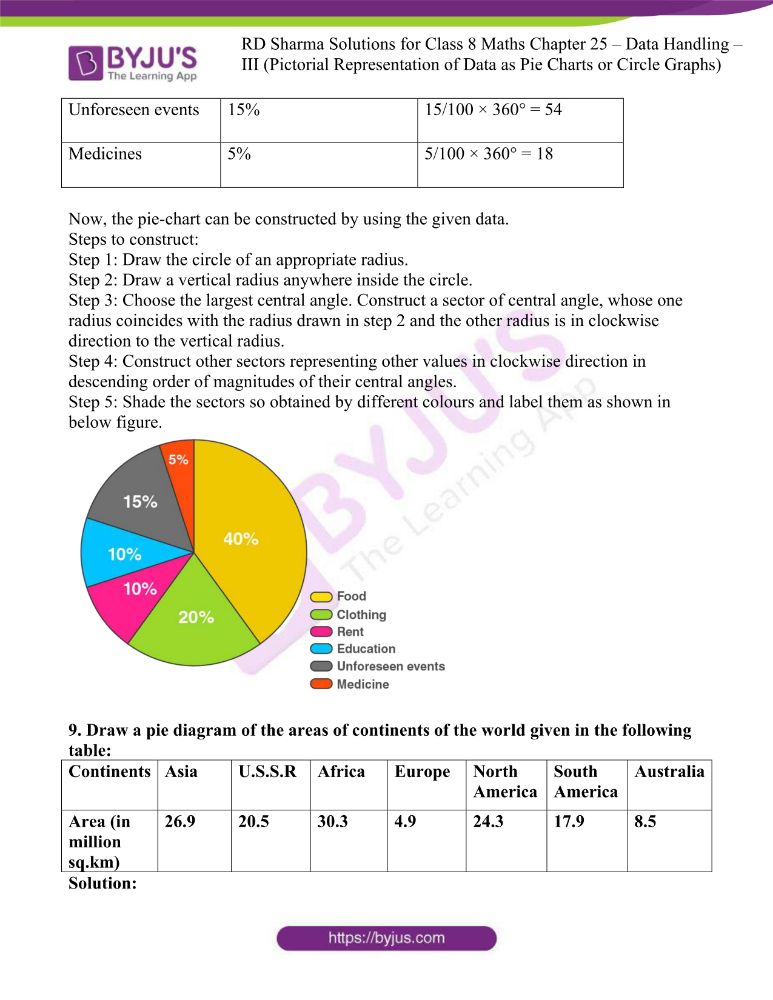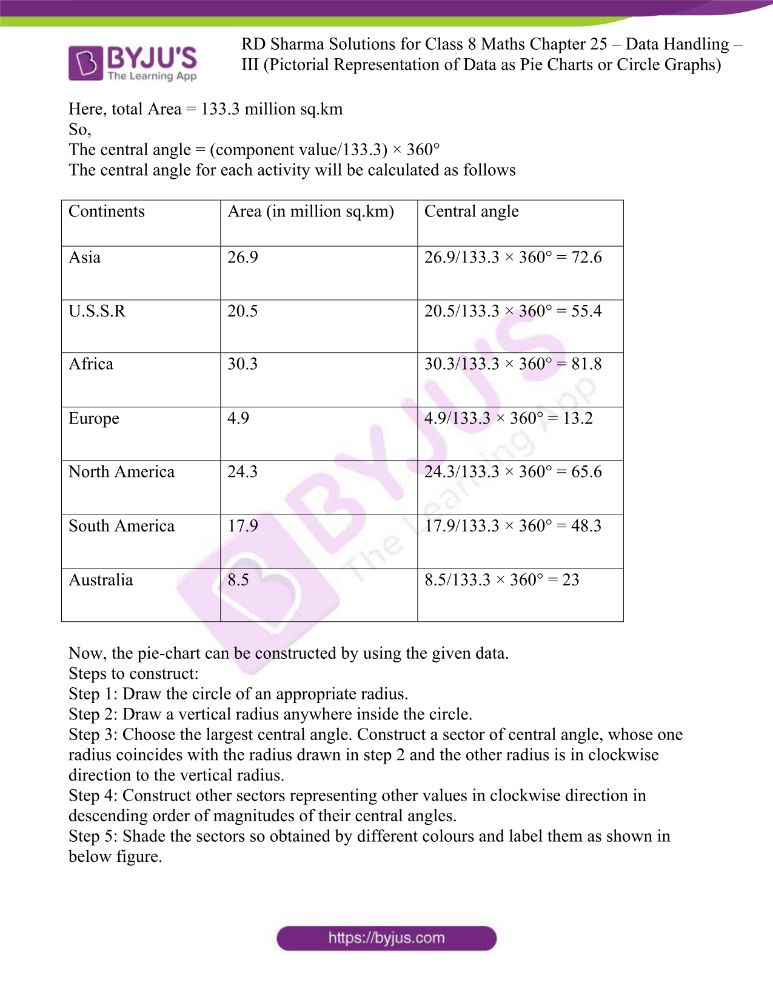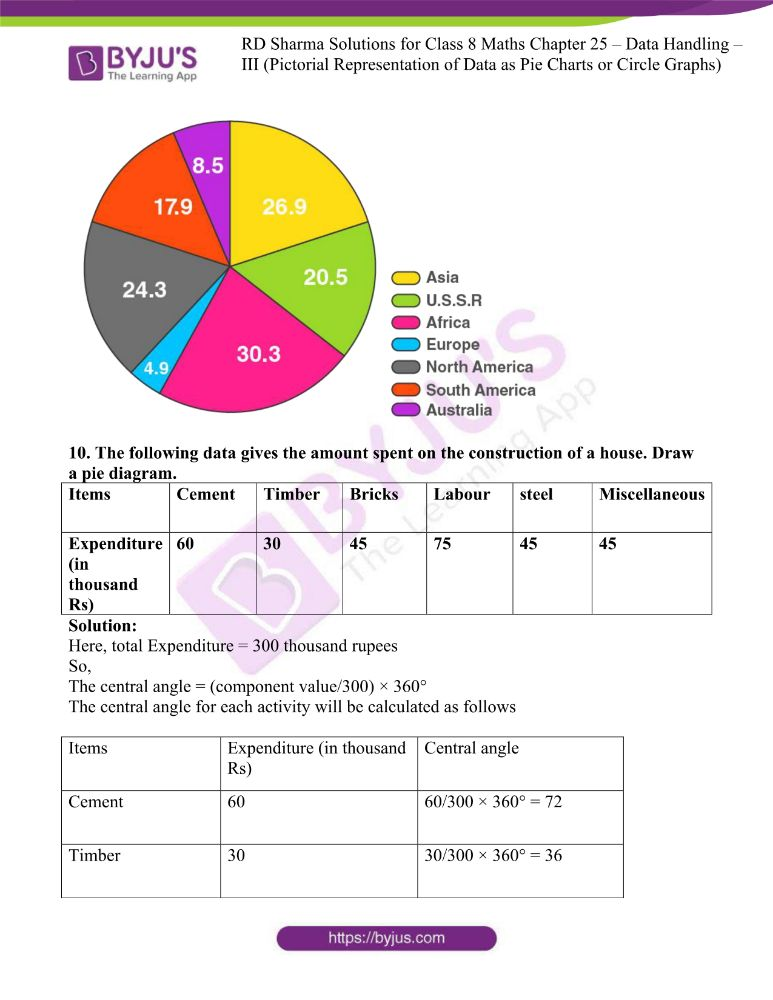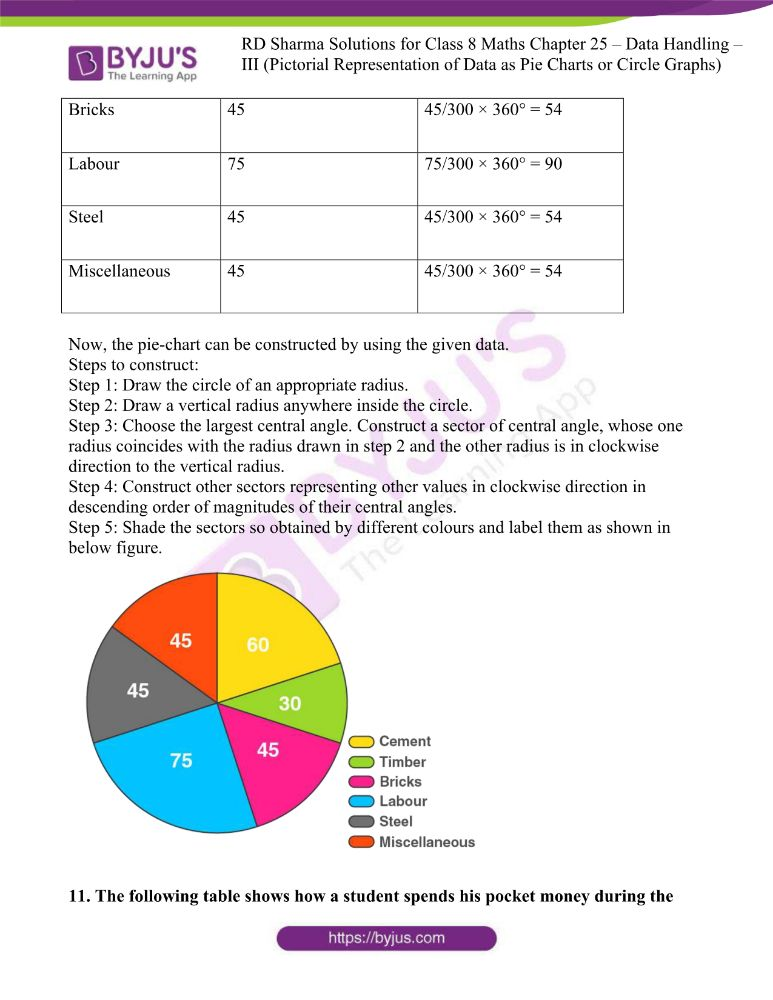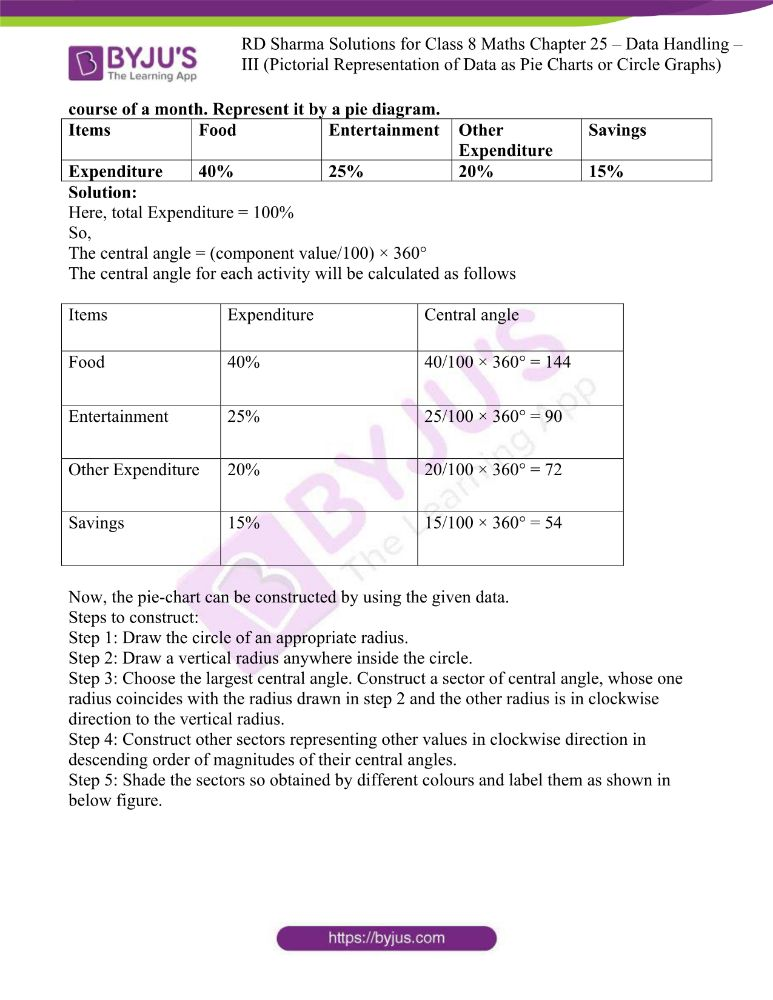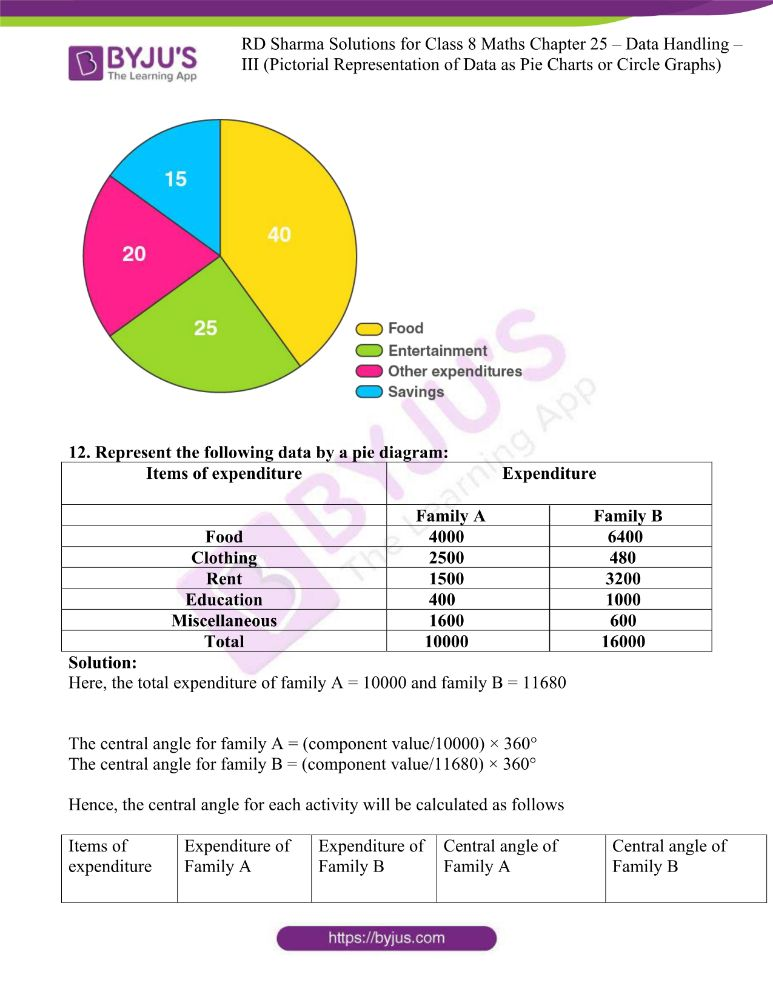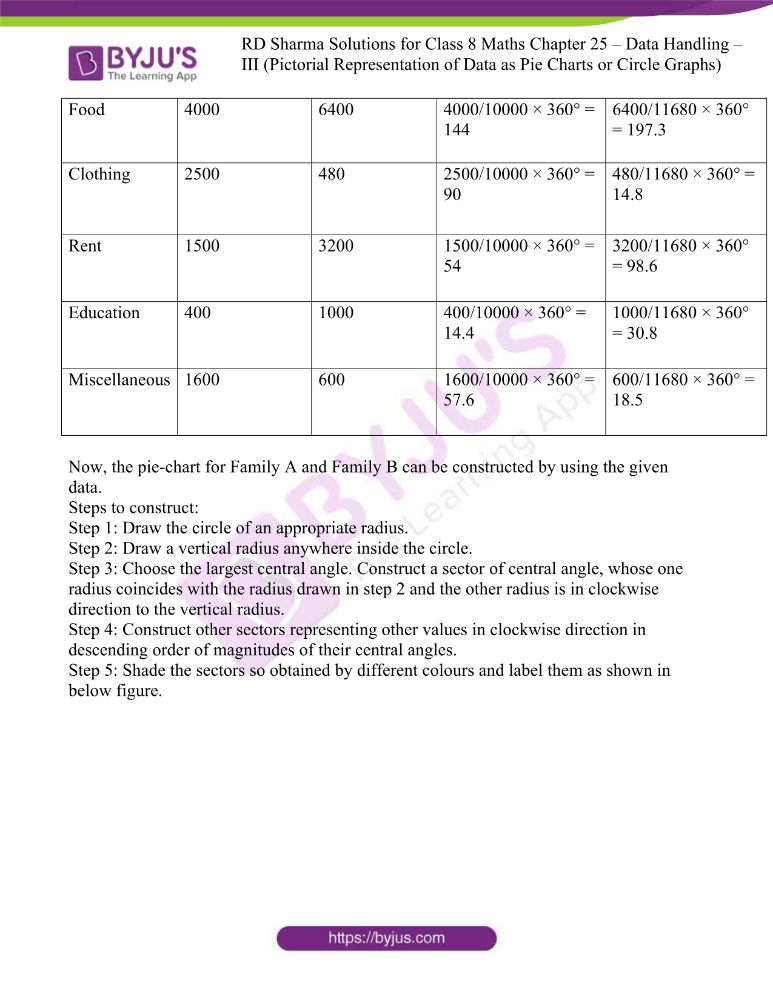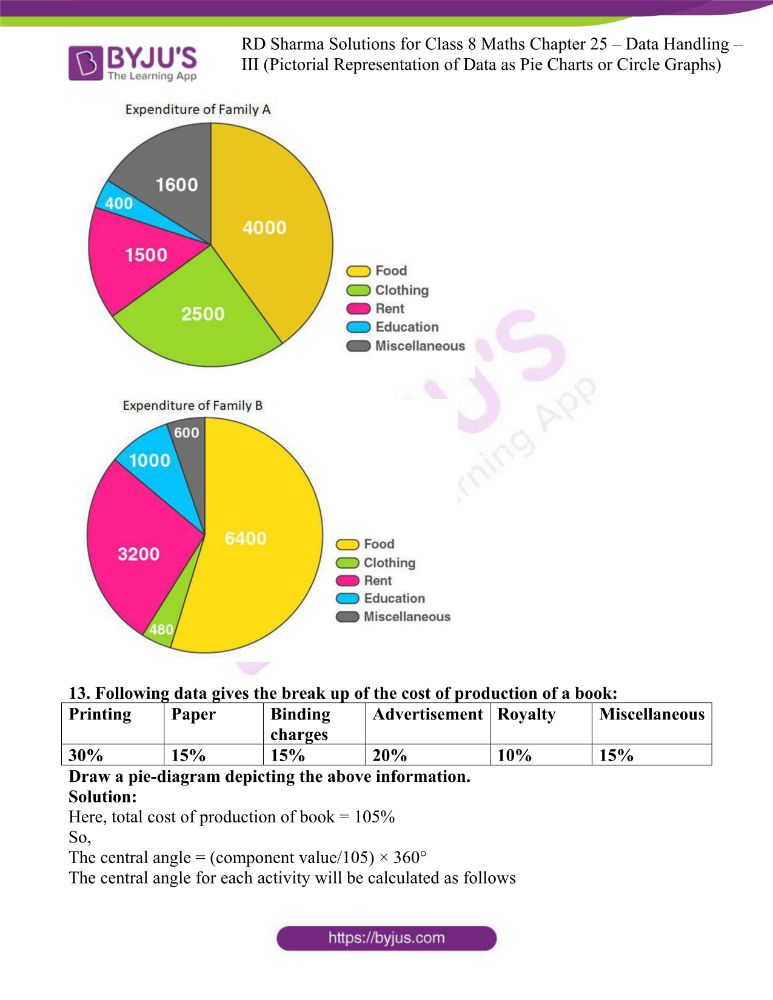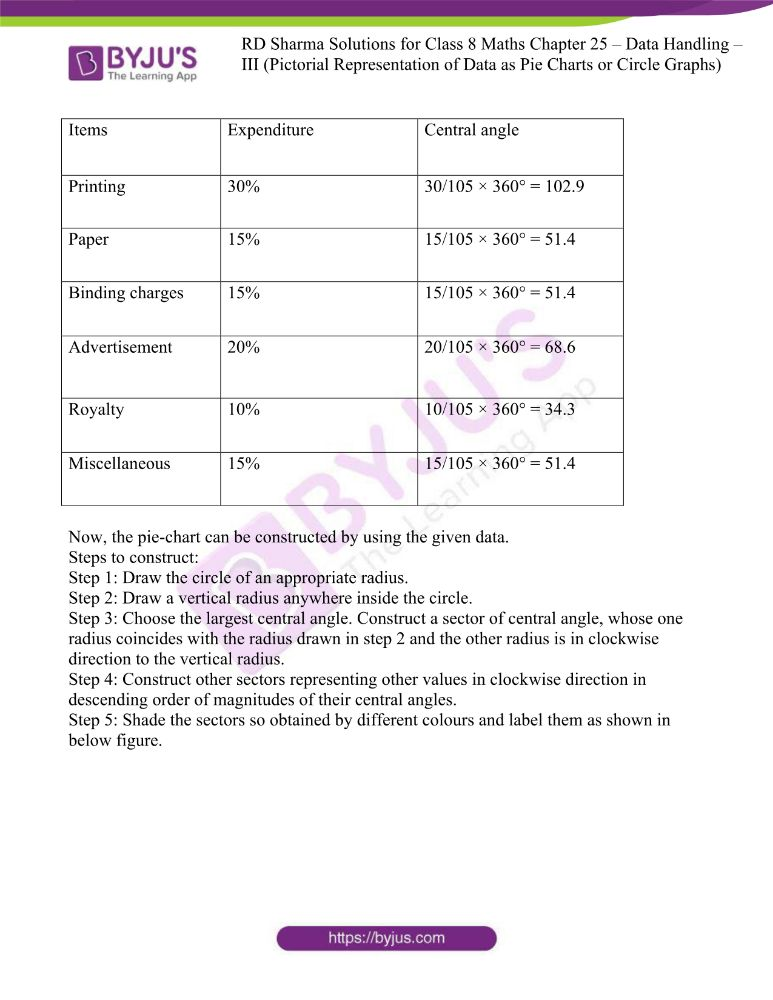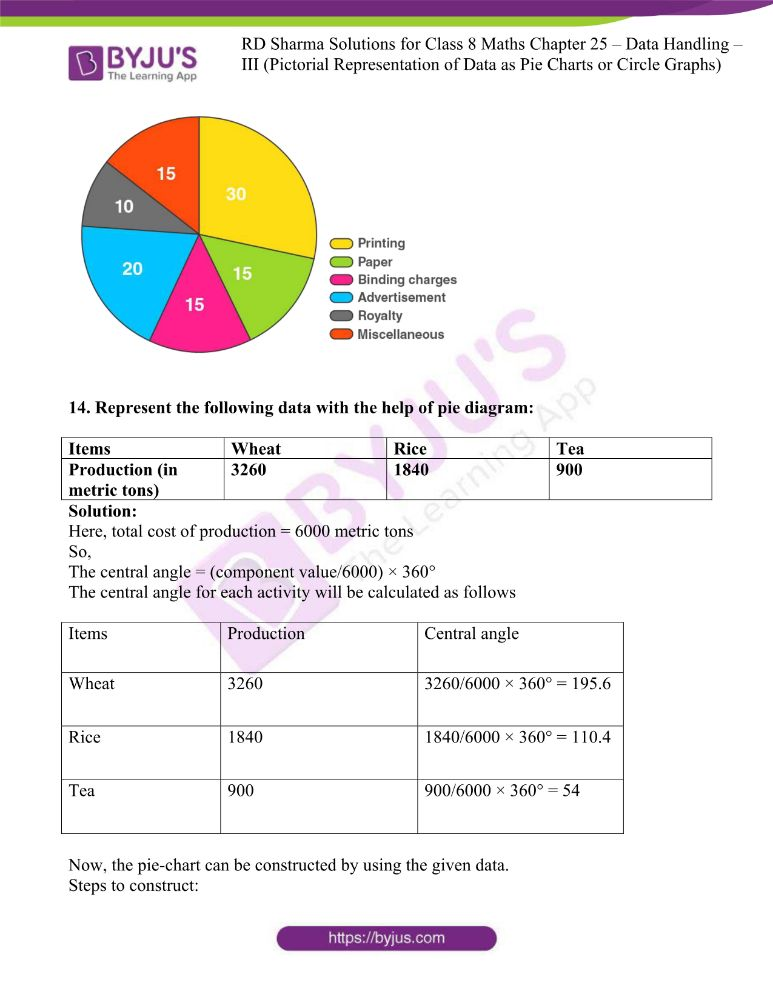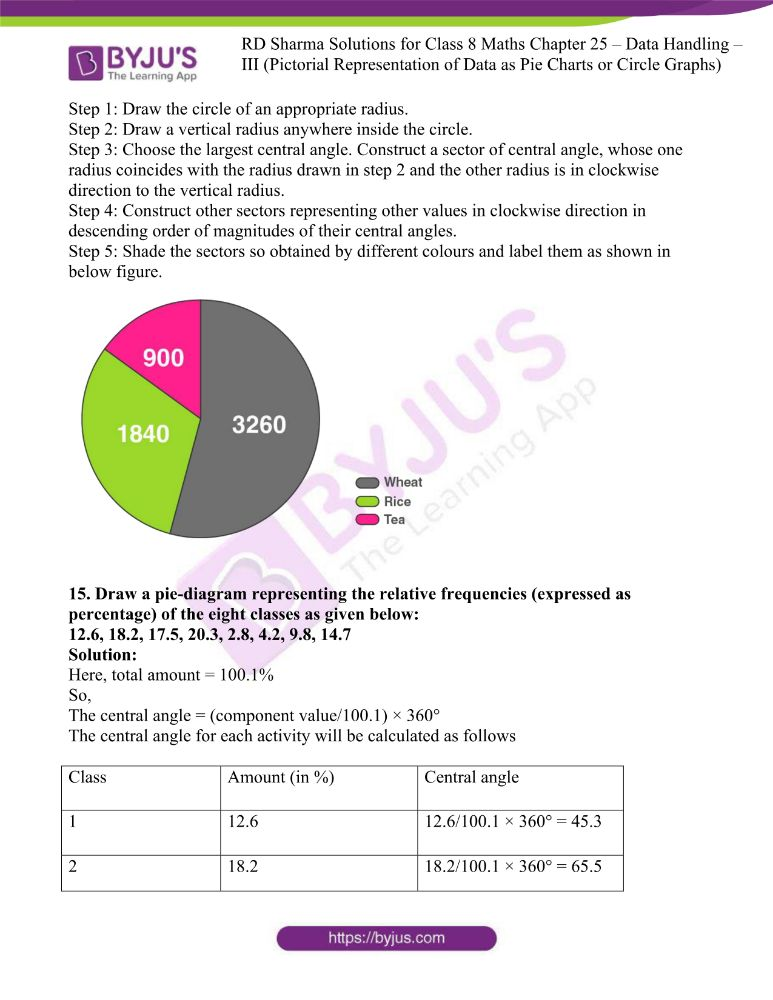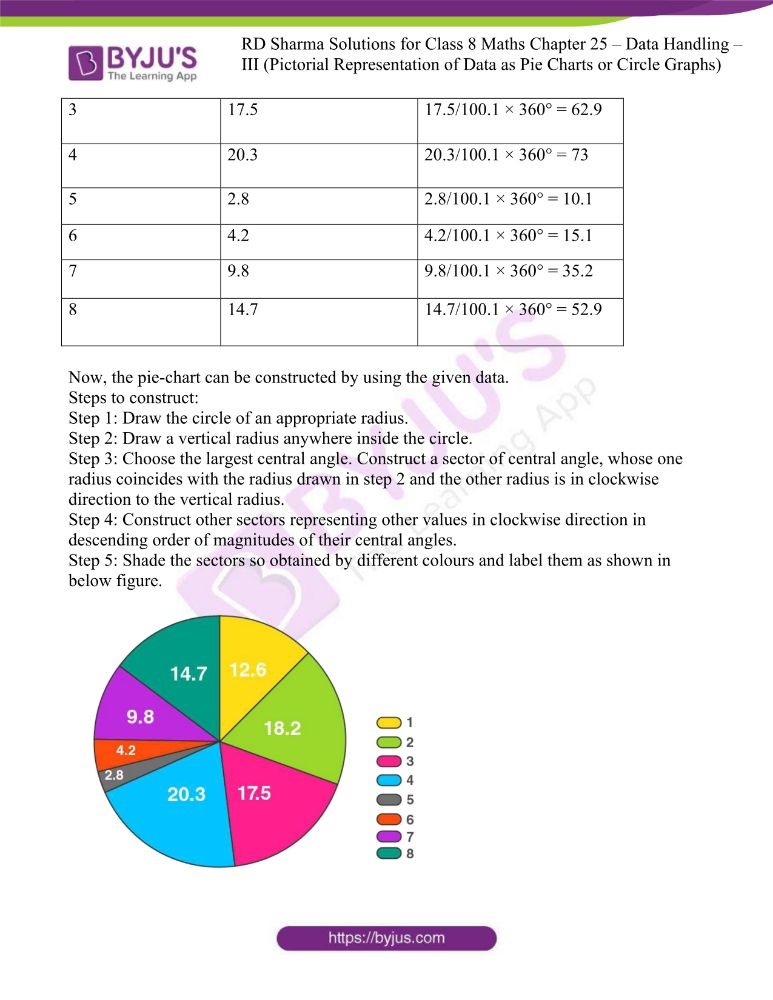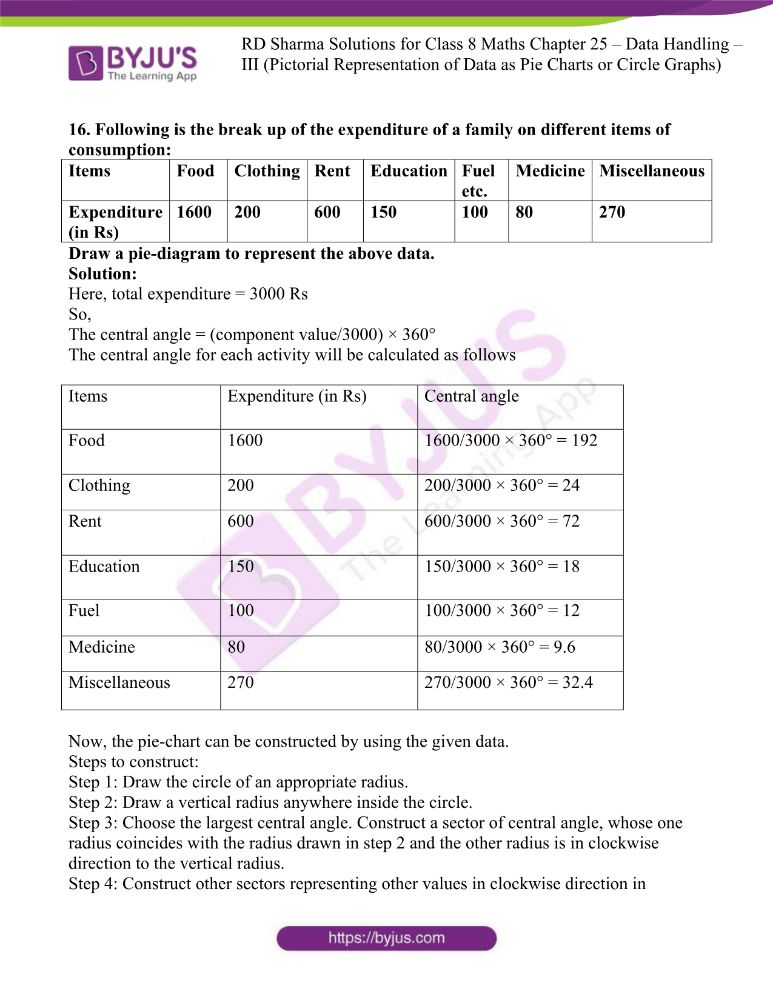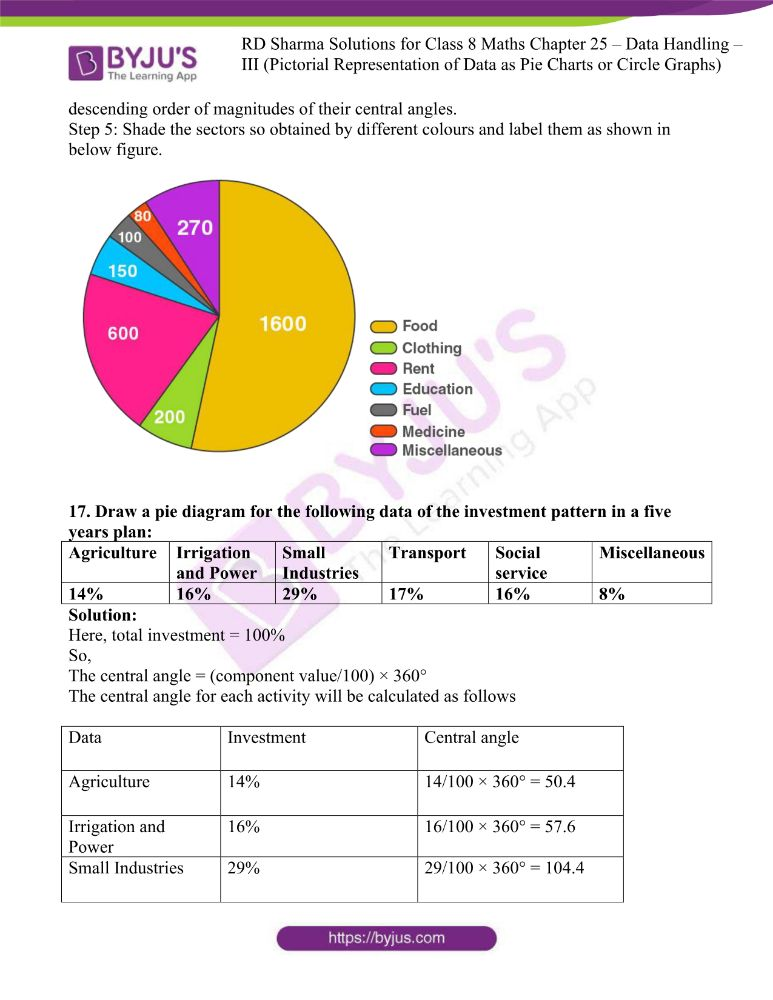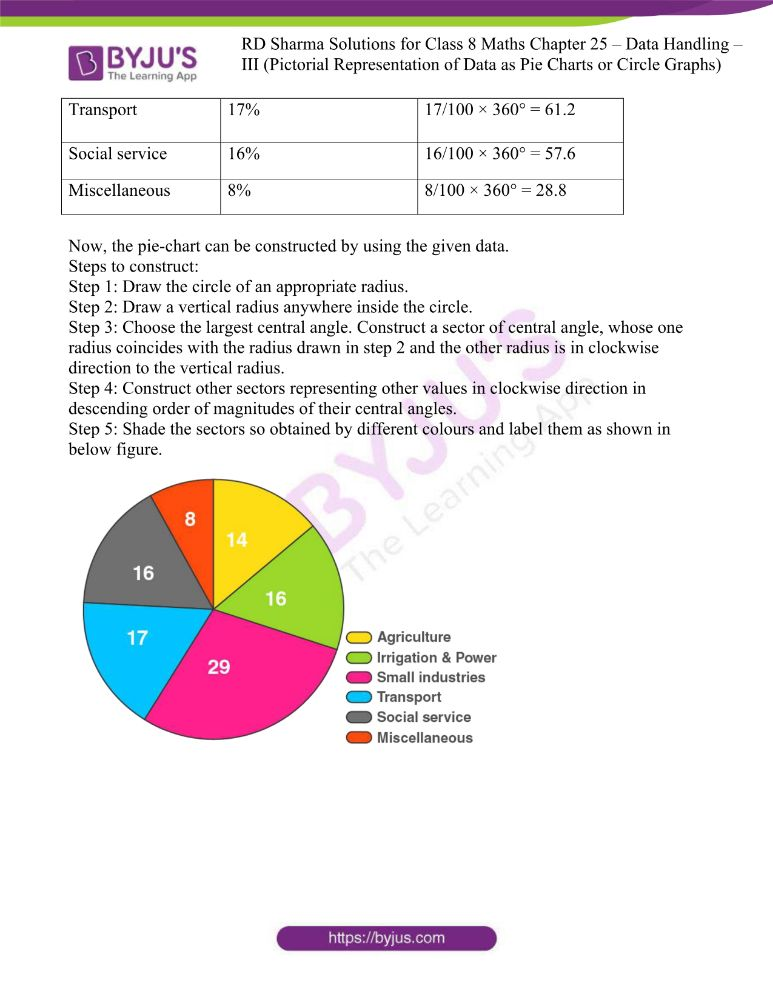### Access answers to RD Sharma Maths Solutions For Class 8 Exercise 25.1 Chapter 25 Data Handling – III (Pictorial Representation of Data as Pie Charts or Circle Graphs)

1. The number of hours, spent by a school boy on different activities in a working day, is given below:

 Activities Sleep School Home Play Others Total Number of Hours 8 7 4 2 3 24

Present the information in the form of a pie-chart.

Solution:

Here, total number of hours = 24

So,

The central angle = (component value/24) × 360°

The central angle for each activity will be calculated as follows

 Activity Number of Hours Central Angle Sleep 8 8/24 × 360° = 120o School 7 7/24 × 360° = 105o Home 4 4/24 × 360° = 60o Play 2 2/24 × 360° = 30o Others 3 3/24 × 360° = 45o

Now, the pie-chart can be constructed by using the given data.

Steps to construct:

Step 1: Draw the circle of an appropriate radius.

Step 2: Draw a vertical radius anywhere inside the circle.

Step 3: Choose the largest central angle. Here, it is 120o. Construct a sector of central angle 120o whose one radius coincides with the radius drawn in step 2 and the other radius is in clockwise direction to the vertical radius.

Step 4: Construct other sectors representing other values in clockwise direction in descending order of magnitudes of their central angles.

Step 5: Shade the sectors so obtained by different colours and label them as shown in below figure.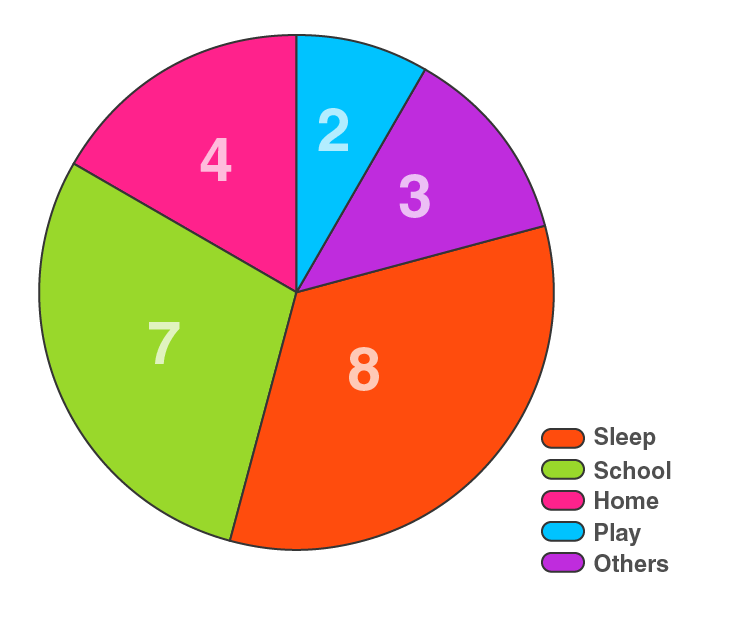2. Employees of a company have been categorized according to their religions as given below:

 Religious Hindu Muslim Sikh Christian Others Total Number of Workers 420 300 225 105 30 1080

Draw a pie-chart to represent the above information.

Solution:

Here, total number of workers = 1080

So,

The central angle = (component value/1080) × 360°

The central angle for each activity will be calculated as follows

 Religious Number of Workers Central Angle Hindu 420 420/1080 × 360° = 144 Muslim 300 300/1080 × 360° = 100 Sikh 225 225/1080 × 360° = 75 Christian 105 105/1080 × 360° = 35 Others 30 30/1080 × 360° = 10

Now, the pie-chart can be constructed by using the given data.

Steps to construct:

Step 1: Draw the circle of an appropriate radius.

Step 2: Draw a vertical radius anywhere inside the circle.

Step 3: Choose the largest central angle. Construct a sector of central angle, whose one radius coincides with the radius drawn in step 2 and the other radius is in clockwise direction to the vertical radius.

Step 4: Construct other sectors representing other values in clockwise direction in descending order of magnitudes of their central angles.

Step 5: Shade the sectors so obtained by different colours and label them as shown in below figure.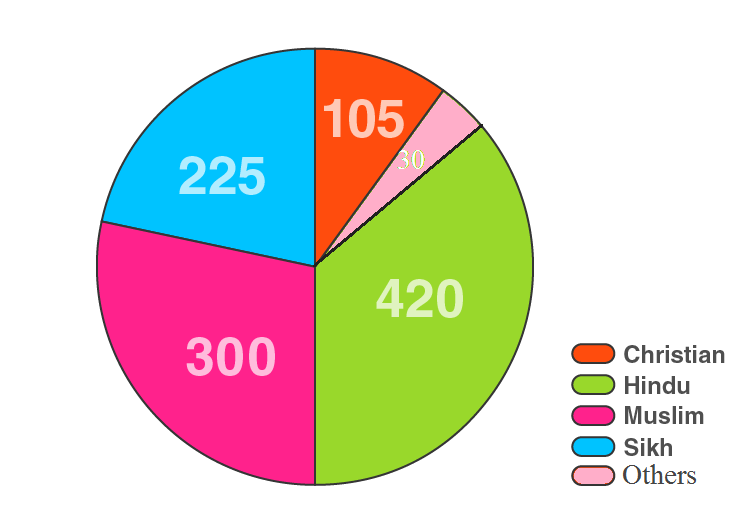3. In one day the sales (in rupees) of different items of a baker’s shop are given below:

 Items Ordinary bread Fruit bread Cakes and Pastries Biscuits Others Total Sales (in Rs) 260 40 100 60 20 480

Draw a pie-chart to represent the above information.

Solution:

Here, total sales = 480

So,

The central angle = (component value/480) × 360°

The central angle for each activity will be calculated as follows

 Items Sales (in Rs) Central Angle Ordinary bread 260 260/480 × 360° = 195 Fruit bread 40 40/480 × 360° = 30 Cakes and Pastries 100 100/480 × 360° = 75 Biscuits 60 60/480 × 360° = 45 Others 20 20/480 × 360° = 15

Now, the pie-chart can be constructed by using the given data.

Steps to construct:

Step 1: Draw the circle of an appropriate radius.

Step 2: Draw a vertical radius anywhere inside the circle.

Step 3: Choose the largest central angle. Construct a sector of central angle, whose one radius coincides with the radius drawn in step 2 and the other radius is in clockwise direction to the vertical radius.

Step 4: Construct other sectors representing other values in clockwise direction in descending order of magnitudes of their central angles.

Step 5: Shade the sectors so obtained by different colours and label them as shown in below figure.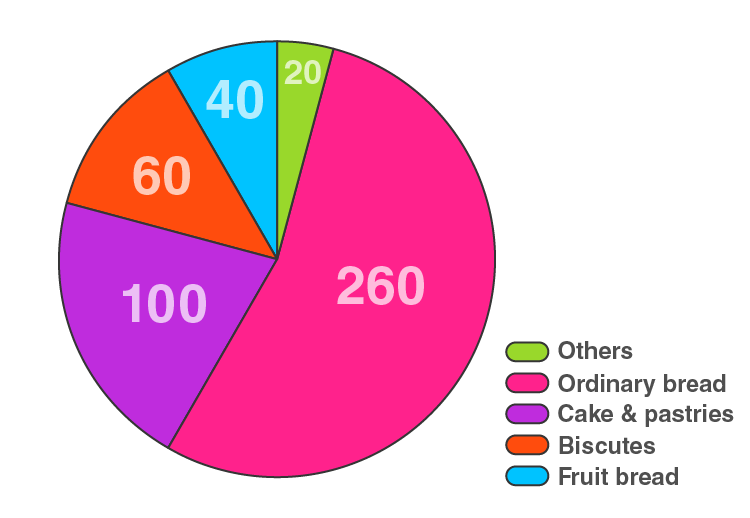4. The following data shows the expenditure of a person on different items during a month. Represent the data by a pie-chart.

 Items of expenditure Rent Education Food Clothing Others Amount (in Rs) 2700 1800 2400 1500 2400

Solution:

Here, total amount = Rs 10800

So,

The central angle = (component value/10800) × 360°

The central angle for each activity will be calculated as follows

 Items of expenditure Amount (in Rs) Central angle Rent 2700 2700/10800 × 360° = 90 Education 1800 1800/10800 × 360° = 60 Food 2400 2400/10800 × 360° = 80 Clothing 1500 1500/10800 × 360° = 50 Others 2400 2400/10800 × 360° = 80

Now, the pie-chart can be constructed by using the given data.

Steps to construct:

Step 1: Draw the circle of an appropriate radius.

Step 2: Draw a vertical radius anywhere inside the circle.

Step 3: Choose the largest central angle. Construct a sector of central angle, whose one radius coincides with the radius drawn in step 2 and the other radius is in clockwise direction to the vertical radius.

Step 4: Construct other sectors representing other values in clockwise direction in descending order of magnitudes of their central angles.

Step 5: Shade the sectors so obtained by different colours and label them as shown in below figure.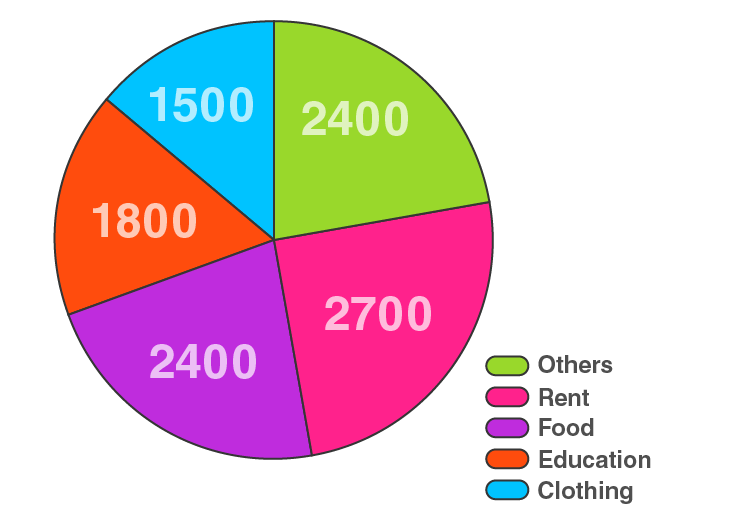5. The percentages of various categories of workers in a state are given in the following table.

 Categories Cultivators Agricultural Labourers Industrial Workers Commercial Workers Others % of workers 40 25 12.5 10 12.5

Present the information in the form of a pie-chart.

Solution:

Here, total % of workers = 100%

So,

The central angle = (component value/100) × 360°

The central angle for each activity will be calculated as follows

 Categories % of workers Central angle Cultivators 40 400/100 × 360° = 144 Agricultural Labourers 25 25/100 × 360° = 90 Industrial Workers 12.5 12.5/100 × 360° = 45 Commercial Workers 10 10/100 × 360° = 36 Others 12.5 12.5/100 × 360° = 45

Now, the pie-chart can be constructed by using the given data.

Steps to construct:

Step 1: Draw the circle of an appropriate radius.

Step 2: Draw a vertical radius anywhere inside the circle.

Step 3: Choose the largest central angle. Construct a sector of central angle, whose one radius coincides with the radius drawn in step 2 and the other radius is in clockwise direction to the vertical radius.

Step 4: Construct other sectors representing other values in clockwise direction in descending order of magnitudes of their central angles.

Step 5: Shade the sectors so obtained by different colours and label them as shown in below figure.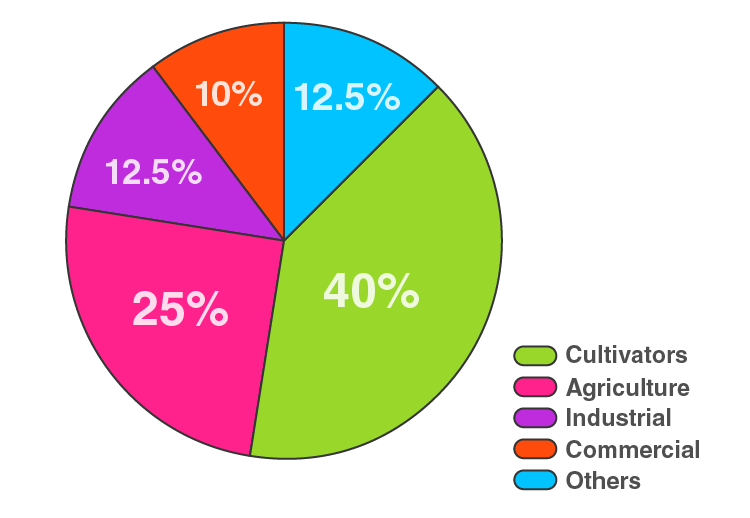6. The following table shows the expenditure incurred by a publisher in publishing a book:

 Items Papers Printing Binding Advertising Miscellaneous Expenditure (in %) 35% 20% 10% 5% 30%

Present the above data in the form of pie-chart.

Solution:

Here, total Expenditure (in %) = 100%

So,

The central angle = (component value/100) × 360°

The central angle for each activity will be calculated as follows

 Items Expenditure (in %) Central angle Papers 35% 35/100 × 360° = 126 Printing 20% 20/100 × 360° = 72 Binding 10% 10/100 × 360° = 36 Advertising 5% 5/100 × 360° = 18 Miscellaneous 30% 30/100 × 360° = 108

Now, the pie-chart can be constructed by using the given data.

Steps to construct:

Step 1: Draw the circle of an appropriate radius.

Step 2: Draw a vertical radius anywhere inside the circle.

Step 3: Choose the largest central angle. Construct a sector of central angle, whose one radius coincides with the radius drawn in step 2 and the other radius is in clockwise direction to the vertical radius.

Step 4: Construct other sectors representing other values in clockwise direction in descending order of magnitudes of their central angles.

Step 5: Shade the sectors so obtained by different colours and label them as shown in below figure.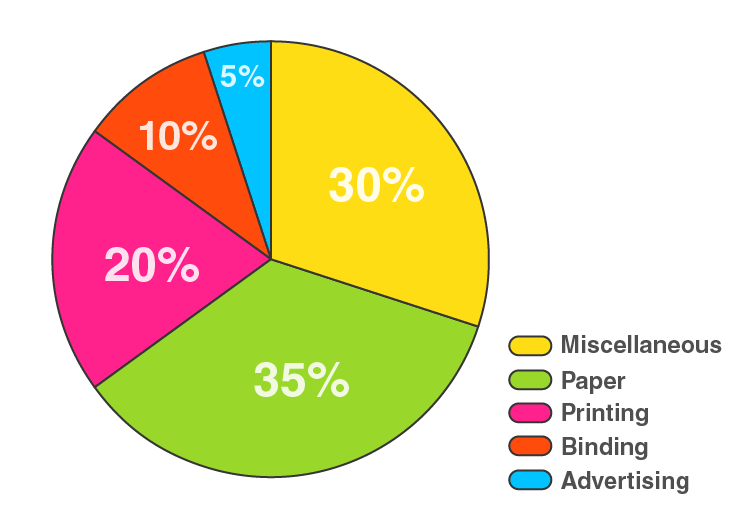7. Percentage of the different products of a village in a particular district are given below. Draw a pie chart representing this information.

 Items Wheat Pulses Jwar Groundnuts Vegetables Total % 125/3 125/6 25/2 50/3 25/3 100

Solution:

Here, total % = 100%

So,

The central angle = (component value/100) × 360°

The central angle for each activity will be calculated as follows

 Items % Central angle Wheat 125/3 (125/3)/100 × 360° = 150 Pulses 125/6 (125/6)/100 × 360° = 75 Jwar 25/2 (25/2)/100 × 360° = 45 Groundnuts 50/3 (50/3)/100 × 360° = 60 Vegetables 25/3 (25/3)/100 × 360° = 30

Now, the pie-chart can be constructed by using the given data.

Steps to construct:

Step 1: Draw the circle of an appropriate radius.

Step 2: Draw a vertical radius anywhere inside the circle.

Step 3: Choose the largest central angle. Construct a sector of central angle, whose one radius coincides with the radius drawn in step 2 and the other radius is in clockwise direction to the vertical radius.

Step 4: Construct other sectors representing other values in clockwise direction in descending order of magnitudes of their central angles.

Step 5: Shade the sectors so obtained by different colours and label them as shown in below figure.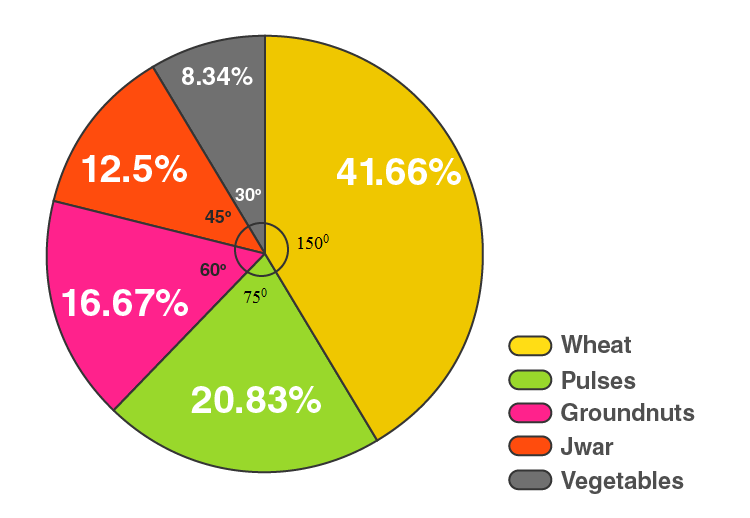8. Draw a pie diagram for the following data of expenditure pattern in a family:

 Items Food Clothing Rent Education Unforeseen events Medicine Expenditure (in %) 40% 20% 10% 10% 15% 5%

Solution:

Here, total % = 100%

So,

The central angle = (component value/100) × 360°

The central angle for each activity will be calculated as follows

 Items Expenditure (in %) Central angle Food 40% 40/100 × 360° = 144 Clothing 20% 20/100 × 360° = 72 Rent 10% 10/100 × 360° = 36 Education 10% 10/100 × 360° = 36 Unforeseen events 15% 15/100 × 360° = 54 Medicines 5% 5/100 × 360° = 18

Now, the pie-chart can be constructed by using the given data.

Steps to construct:

Step 1: Draw the circle of an appropriate radius.

Step 2: Draw a vertical radius anywhere inside the circle.

Step 3: Choose the largest central angle. Construct a sector of central angle, whose one radius coincides with the radius drawn in step 2 and the other radius is in clockwise direction to the vertical radius.

Step 4: Construct other sectors representing other values in clockwise direction in descending order of magnitudes of their central angles.

Step 5: Shade the sectors so obtained by different colours and label them as shown in below figure.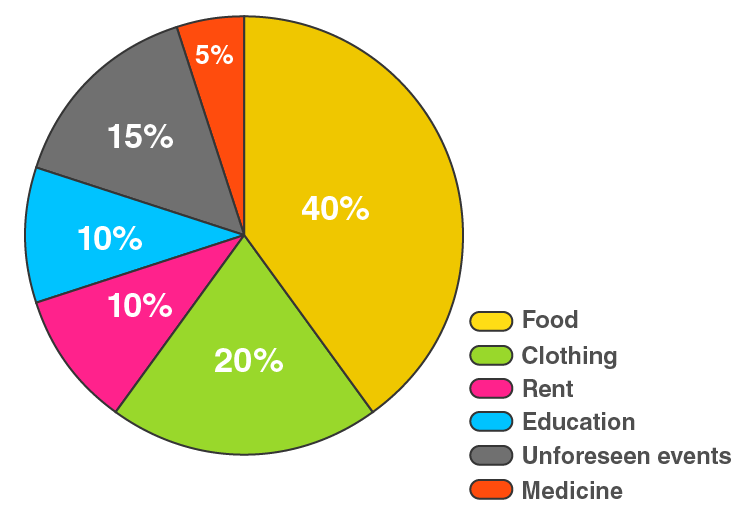9. Draw a pie diagram of the areas of continents of the world given in the following table:

 Continents Asia U.S.S.R Africa Europe North America South America Australia Area (in million sq.km) 26.9 20.5 30.3 4.9 24.3 17.9 8.5

Solution:

Here, total Area = 133.3 million sq.km

So,

The central angle = (component value/133.3) × 360°

The central angle for each activity will be calculated as follows

 Continents Area (in million sq.km) Central angle Asia 26.9 26.9/133.3 × 360° = 72.6 U.S.S.R 20.5 20.5/133.3 × 360° = 55.4 Africa 30.3 30.3/133.3 × 360° = 81.8 Europe 4.9 4.9/133.3 × 360° = 13.2 North America 24.3 24.3/133.3 × 360° = 65.6 South America 17.9 17.9/133.3 × 360° = 48.3 Australia 8.5 8.5/133.3 × 360° = 23

Now, the pie-chart can be constructed by using the given data.

Steps to construct:

Step 1: Draw the circle of an appropriate radius.

Step 2: Draw a vertical radius anywhere inside the circle.

Step 3: Choose the largest central angle. Construct a sector of central angle, whose one radius coincides with the radius drawn in step 2 and the other radius is in clockwise direction to the vertical radius.

Step 4: Construct other sectors representing other values in clockwise direction in descending order of magnitudes of their central angles.

Step 5: Shade the sectors so obtained by different colours and label them as shown in below figure.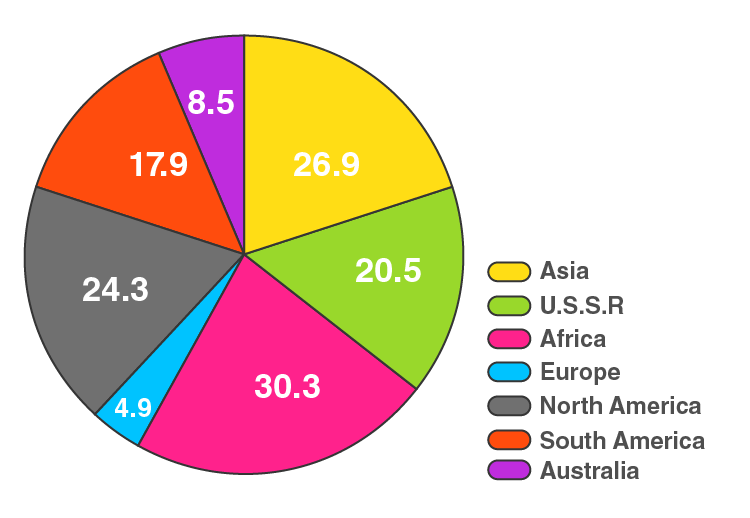10. The following data gives the amount spent on the construction of a house. Draw a pie diagram.

 Items Cement Timber Bricks Labour steel Miscellaneous Expenditure (in thousand Rs) 60 30 45 75 45 45

Solution:

Here, total Expenditure = 300 thousand rupees

So,

The central angle = (component value/300) × 360°

The central angle for each activity will be calculated as follows

 Items Expenditure (in thousand Rs) Central angle Cement 60 60/300 × 360° = 72 Timber 30 30/300 × 360° = 36 Bricks 45 45/300 × 360° = 54 Labour 75 75/300 × 360° = 90 Steel 45 45/300 × 360° = 54 Miscellaneous 45 45/300 × 360° = 54

Now, the pie-chart can be constructed by using the given data.

Steps to construct:

Step 1: Draw the circle of an appropriate radius.

Step 2: Draw a vertical radius anywhere inside the circle.

Step 3: Choose the largest central angle. Construct a sector of central angle, whose one radius coincides with the radius drawn in step 2 and the other radius is in clockwise direction to the vertical radius.

Step 4: Construct other sectors representing other values in clockwise direction in descending order of magnitudes of their central angles.

Step 5: Shade the sectors so obtained by different colours and label them as shown in below figure.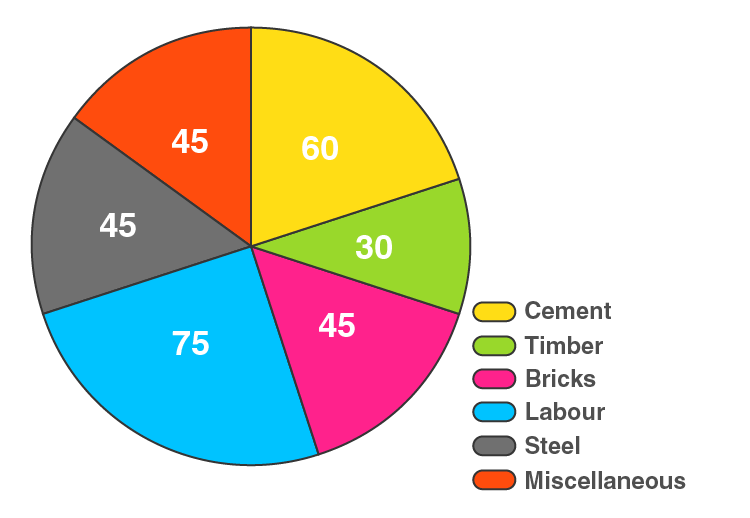11. The following table shows how a student spends his pocket money during the course of a month. Represent it by a pie diagram.

 Items Food Entertainment Other Expenditure Savings Expenditure 40% 25% 20% 15%

Solution:

Here, total Expenditure = 100%

So,

The central angle = (component value/100) × 360°

The central angle for each activity will be calculated as follows

 Items Expenditure Central angle Food 40% 40/100 × 360° = 144 Entertainment 25% 25/100 × 360° = 90 Other Expenditure 20% 20/100 × 360° = 72 Savings 15% 15/100 × 360° = 54

Now, the pie-chart can be constructed by using the given data.

Steps to construct:

Step 1: Draw the circle of an appropriate radius.

Step 2: Draw a vertical radius anywhere inside the circle.

Step 3: Choose the largest central angle. Construct a sector of central angle, whose one radius coincides with the radius drawn in step 2 and the other radius is in clockwise direction to the vertical radius.

Step 4: Construct other sectors representing other values in clockwise direction in descending order of magnitudes of their central angles.

Step 5: Shade the sectors so obtained by different colours and label them as shown in below figure.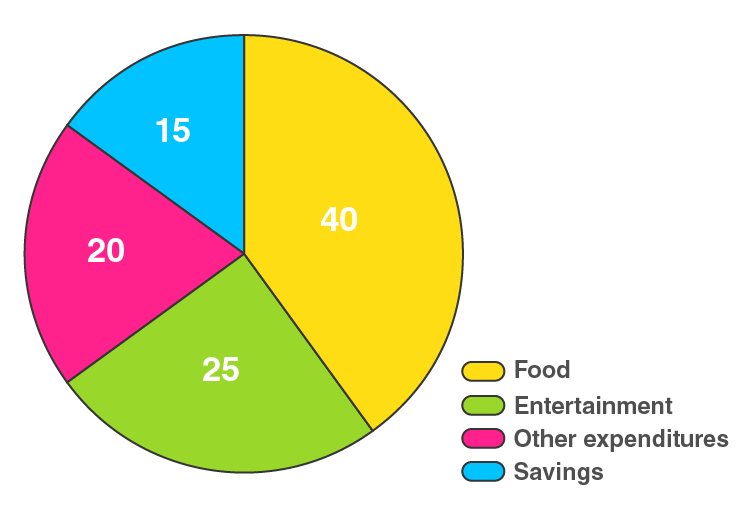12. Represent the following data by a pie diagram:

 Items of expenditure Expenditure Family A Family B Food 4000 6400 Clothing 2500 480 Rent 1500 3200 Education 400 1000 Miscellaneous 1600 600 Total 10000 16000

Solution:

Here, the total expenditure of family A = 10000 and family B = 11680

The central angle for family A = (component value/10000) × 360°

The central angle for family B = (component value/11680) × 360°

Hence, the central angle for each activity will be calculated as follows

 Items of expenditure Expenditure of Family A Expenditure of Family B Central angle of Family A Central angle of Family B Food 4000 6400 4000/10000 × 360° = 144 6400/11680 × 360° = 197.3 Clothing 2500 480 2500/10000 × 360° = 90 480/11680 × 360° = 14.8 Rent 1500 3200 1500/10000 × 360° = 54 3200/11680 × 360° = 98.6 Education 400 1000 400/10000 × 360° = 14.4 1000/11680 × 360° = 30.8 Miscellaneous 1600 600 1600/10000 × 360° = 57.6 600/11680 × 360° = 18.5

Now, the pie-chart for Family A and Family B can be constructed by using the given data.

Steps to construct:

Step 1: Draw the circle of an appropriate radius.

Step 2: Draw a vertical radius anywhere inside the circle.

Step 3: Choose the largest central angle. Construct a sector of central angle, whose one radius coincides with the radius drawn in step 2 and the other radius is in clockwise direction to the vertical radius.

Step 4: Construct other sectors representing other values in clockwise direction in descending order of magnitudes of their central angles.

Step 5: Shade the sectors so obtained by different colours and label them as shown in below figure.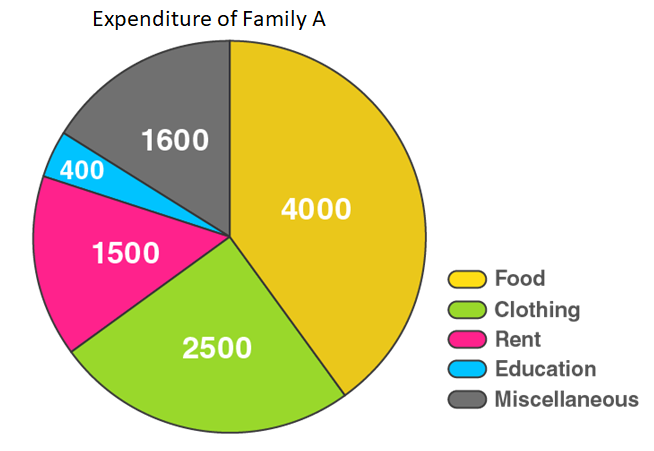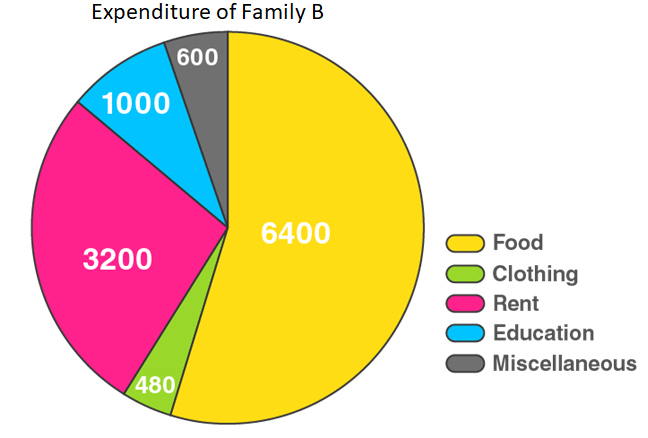13. Following data gives the break up of the cost of production of a book:

 Printing Paper Binding charges Advertisement Royalty Miscellaneous 30% 15% 15% 20% 10% 15%

Draw a pie-diagram depicting the above information.

Solution:

Here, total cost of production of book = 105%

So,

The central angle = (component value/105) × 360°

The central angle for each activity will be calculated as follows

 Items Expenditure Central angle Printing 30% 30/105 × 360° = 102.9 Paper 15% 15/105 × 360° = 51.4 Binding charges 15% 15/105 × 360° = 51.4 Advertisement 20% 20/105 × 360° = 68.6 Royalty 10% 10/105 × 360° = 34.3 Miscellaneous 15% 15/105 × 360° = 51.4

Now, the pie-chart can be constructed by using the given data.

Steps to construct:

Step 1: Draw the circle of an appropriate radius.

Step 2: Draw a vertical radius anywhere inside the circle.

Step 3: Choose the largest central angle. Construct a sector of central angle, whose one radius coincides with the radius drawn in step 2 and the other radius is in clockwise direction to the vertical radius.

Step 4: Construct other sectors representing other values in clockwise direction in descending order of magnitudes of their central angles.

Step 5: Shade the sectors so obtained by different colours and label them as shown in below figure.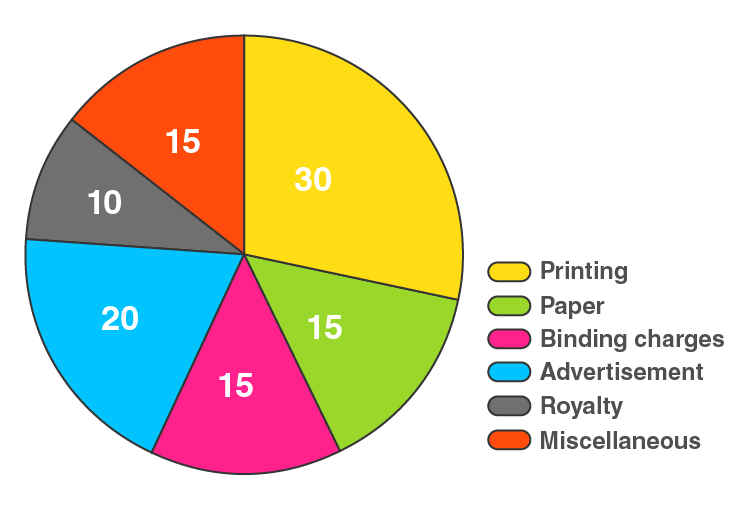14. Represent the following data with the help of pie diagram:

 Items Wheat Rice Tea Production (in metric tons) 3260 1840 900

Solution:

Here, total cost of production = 6000 metric tons

So,

The central angle = (component value/6000) × 360°

The central angle for each activity will be calculated as follows

 Items Production Central angle Wheat 3260 3260/6000 × 360° = 195.6 Rice 1840 1840/6000 × 360° = 110.4 Tea 900 900/6000 × 360° = 54

Now, the pie-chart can be constructed by using the given data.

Steps to construct:

Step 1: Draw the circle of an appropriate radius.

Step 2: Draw a vertical radius anywhere inside the circle.

Step 3: Choose the largest central angle. Construct a sector of central angle, whose one radius coincides with the radius drawn in step 2 and the other radius is in clockwise direction to the vertical radius.

Step 4: Construct other sectors representing other values in clockwise direction in descending order of magnitudes of their central angles.

Step 5: Shade the sectors so obtained by different colours and label them as shown in below figure.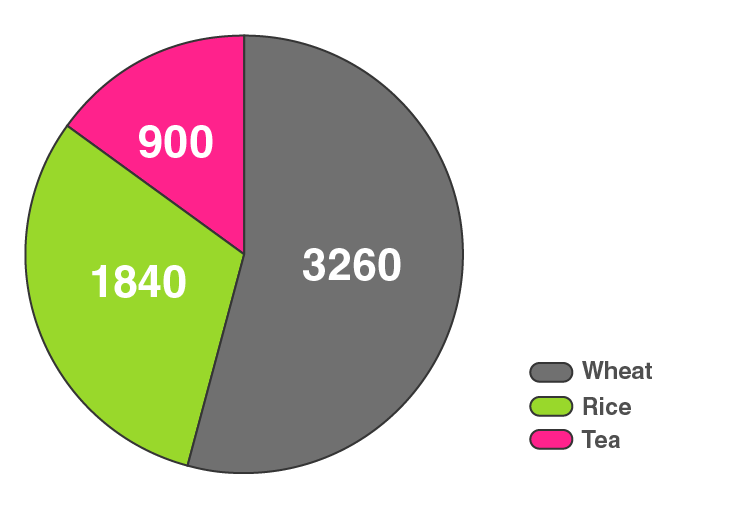15. Draw a pie-diagram representing the relative frequencies (expressed as percentage) of the eight classes as given below:
12.6, 18.2, 17.5, 20.3, 2.8, 4.2, 9.8, 14.7

Solution:

Here, total amount = 100.1%

So,

The central angle = (component value/100.1) × 360°

The central angle for each activity will be calculated as follows

 Class Amount (in %) Central angle 1 12.6 12.6/100.1 × 360° = 45.3 2 18.2 18.2/100.1 × 360° = 65.5 3 17.5 17.5/100.1 × 360° = 62.9 4 20.3 20.3/100.1 × 360° = 73 5 2.8 2.8/100.1 × 360° = 10.1 6 4.2 4.2/100.1 × 360° = 15.1 7 9.8 9.8/100.1 × 360° = 35.2 8 14.7 14.7/100.1 × 360° = 52.9

Now, the pie-chart can be constructed by using the given data.

Steps to construct:

Step 1: Draw the circle of an appropriate radius.

Step 2: Draw a vertical radius anywhere inside the circle.

Step 3: Choose the largest central angle. Construct a sector of central angle, whose one radius coincides with the radius drawn in step 2 and the other radius is in clockwise direction to the vertical radius.

Step 4: Construct other sectors representing other values in clockwise direction in descending order of magnitudes of their central angles.

Step 5: Shade the sectors so obtained by different colours and label them as shown in below figure.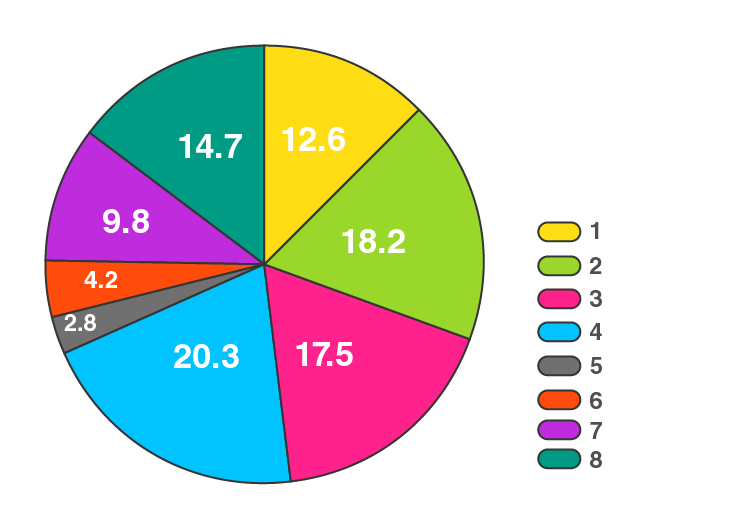16. Following is the break up of the expenditure of a family on different items of consumption:

 Items Food Clothing Rent Education Fuel etc. Medicine Miscellaneous Expenditure (in Rs) 1600 200 600 150 100 80 270

Draw a pie-diagram to represent the above data.

Solution:

Here, total expenditure = 3000 Rs

So,

The central angle = (component value/3000) × 360°

The central angle for each activity will be calculated as follows

 Items Expenditure (in Rs) Central angle Food 1600 1600/3000 × 360° = 192 Clothing 200 200/3000 × 360° = 24 Rent 600 600/3000 × 360° = 72 Education 150 150/3000 × 360° = 18 Fuel 100 100/3000 × 360° = 12 Medicine 80 80/3000 × 360° = 9.6 Miscellaneous 270 270/3000 × 360° = 32.4

Now, the pie-chart can be constructed by using the given data.

Steps to construct:

Step 1: Draw the circle of an appropriate radius.

Step 2: Draw a vertical radius anywhere inside the circle.

Step 3: Choose the largest central angle. Construct a sector of central angle, whose one radius coincides with the radius drawn in step 2 and the other radius is in clockwise direction to the vertical radius.

Step 4: Construct other sectors representing other values in clockwise direction in descending order of magnitudes of their central angles.

Step 5: Shade the sectors so obtained by different colours and label them as shown in below figure.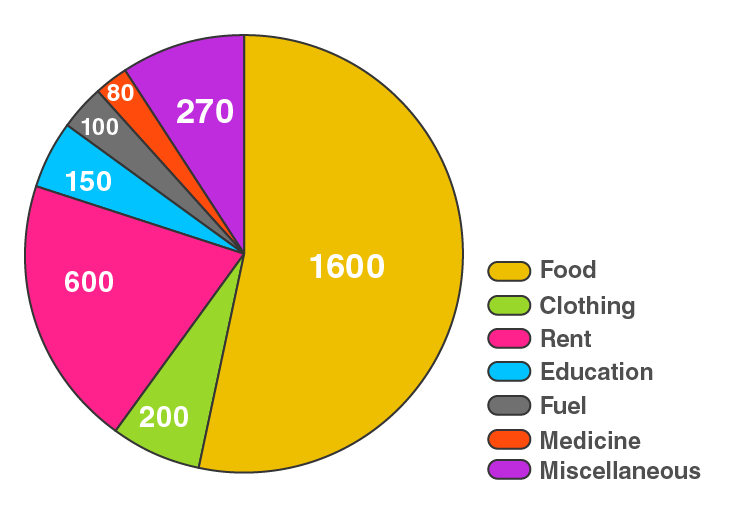17. Draw a pie diagram for the following data of the investment pattern in a five years plan:

 Agriculture Irrigation and Power Small Industries Transport Social service Miscellaneous 14% 16% 29% 17% 16% 8%

Solution:

Here, total investment = 100%

So,

The central angle = (component value/100) × 360°

The central angle for each activity will be calculated as follows

 Data Investment Central angle Agriculture 14% 14/100 × 360° = 50.4 Irrigation and Power 16% 16/100 × 360° = 57.6 Small Industries 29% 29/100 × 360° = 104.4 Transport 17% 17/100 × 360° = 61.2 Social service 16% 16/100 × 360° = 57.6 Miscellaneous 8% 8/100 × 360° = 28.8

Now, the pie-chart can be constructed by using the given data.

Steps to construct:

Step 1: Draw the circle of an appropriate radius.

Step 2: Draw a vertical radius anywhere inside the circle.

Step 3: Choose the largest central angle. Construct a sector of central angle, whose one radius coincides with the radius drawn in step 2 and the other radius is in clockwise direction to the vertical radius.

Step 4: Construct other sectors representing other values in clockwise direction in descending order of magnitudes of their central angles.

Step 5: Shade the sectors so obtained by different colours and label them as shown in below figure.